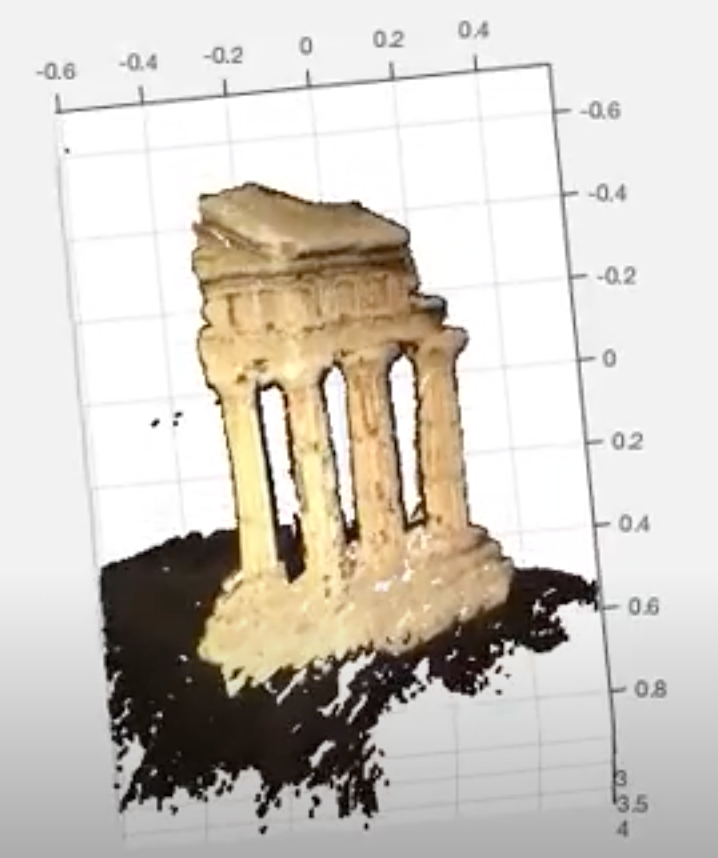CMU

## 3D reconstructionThis is an assignment from CMU 16720-A. In this assignment, we are going to use eight point algorithm to find the Fundamental Matrix (F) from two images. With this F, we can generate the Essential Matrix E if we have the intrinsic camera matrix K1 and K2 for both cameras. Then, we use SVD to find the extrinsic matrix. With both intrinsic and extrinsic matrix, we now have the camera matrix which could be used to do the triangulation. Until now, we have already been able to construct a 3D model from two images. Finally, we will use RANSAC and bundle adjustment to make the result better.

## Eight point algorithm

Since the F matrix is a 3×3 matrix, there are 9 parameters with an arbitrary scale factor. In this case, we need eight correspondences to generate eight equations to find the solution. However, here we “at least” need eight correspondences. If we can have more, it is better. So, to implement this algorithm, we first collect correspondences from two images, and then we scale those positions into the range 0~1. Then, we use SVD to find a 3×3 matrix F. Then, we “unscale” the F. Below is a demo that we use this F to find the epipolar line corresponding to those points we pick.

As you can see, the correspondences (right image) correspond to the points (left image) are all on the epipolar lines, which means our F is good.

## Triangulation

Since we already have the F matrix, and we are also given the camera intrinsic matrix K1 and K2, we can find the essential matrix E. By using this E, we can find 4 possible extrinsic matrix M2s if we fix our M1 to be [I,0]. We can feed those M2s into triangulation and see if the 3D points make sense or not. We want to find the M2 which generate points in front of both cameras.

Reference: CMU 16720-A slides

Now, we can use the two positions in a correspondence and their camera matrixes, with SVD again, to get the 3D coordinate. Below is the example of the point cloud of the temple images we show above.

I also compute every x,y image pair in the image and do the same triangulation to build the dense point cloud.